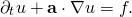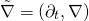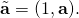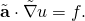# AIR for a space-time hybridizable discontinuous Galerkin method

Abdullah Ali Sivas (University of Waterloo)
Ben Southworth (University of Colorado, Boulder)
Sander Rhebergen (University of Waterloo)

Space-time finite element methods are excellent for the discretization of partial differential equations (PDEs), including on time-dependent domains. Unlike classical time-stepping methods, such as Runge-Kutta or multistep methods, space-time methods make no distinction between spatial and temporal variables. Instead, the PDE is discretized directly in d+1-dimensional space-time, where d is the spatial dimension. Consider, for example, the time-dependent advection equation in d spatial dimensions,To apply the space-time finite element method, we introduce first the space-time gradient